# Single Tile Scenery Elements

The following are the codes for scentery items which occupy a single tile.

Tthe number in brackets [] is the height of the element in decimal. A "Q" indicates that the element occupies only one quadrant of a tile.

00 = Tree 
01 = Tree 
02 = Tree 
03 = Tree 
04 = Tree 
05 = Tree 
06 = Tree 
07 = Tree 

 tree: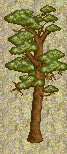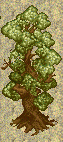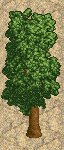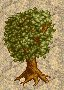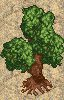code: 00 01 02 03 04 05 06 07

08 = tree  Q
09 = tree  Q
0A = tree  Q
0B = ornamental tree- teardrop  Q
0C = ornamental tree- ball  Q
0D = ornamental tree- cone  Q
0E = ornamental tree- duck  Q
0F = Incense Cedar 
10 = Lawson Cypress  Q
11 = Montery Cypress 
12 = Monkey Puzzle 
13 = Italian Cypress 
14 = Golden Hinoki Cypress 
15 = Arizona Cypress  Q
16 = Hiba 
17 = Common Juniper  Q
18 = Meyer's Blue Juniper 
19 = Caucasion Fir 
1A = Cedar of Lebanon 
1B = Red Fir (large) 
1C = Red Fir (small)  Q
1D = European Larch 
1E = Aleppo Pine 
1F = Scots Pine 
20 = Montezuma Pine 
21 = Corsican Pine 
22 = Black Poplar 
23 = Lombardy Poplar  Q
24 = White Poplar 
25 = Aspen 
26 = Magnolia 
27 = Weeping Willow 
28 = Silver Birch 
29 = Voss' Laburnaw 
2A = Cabbage Tree 
2B = Elephant Fountain 
2C = ornamental tree- gator  Q
2D = ornamental tree- squirell  Q
2E = ornamental tree- heart  Q
2F = ornamental tree- club Q
30 = ornamental tree- diamond Q
31 = ornamental tree- spade Q
32 = Giraffe statue 
33 = Unicorn statue 
34 = Cannary Palm 
35 = Cactus (large) 
36 = Palm tree (tall) 
37 = Palm tree 
38 = Cactus (small)  Q
39 = Garden (large)  Q
3A = Geometric Fountain 
3B = bush  Q
3C = shrub  Q
3D = bush  Q
3E = bush  Q
3F = shrub  Q
40 = bush  Q
41 = Garden (large) 
42 = Garden (large) 
43 = Garden (large) 
44 = Garden (large) 
45 = Garden (large) 
46 = Garden (large) 
47 = Garden (large) 
48 = Garden (large) 
49 = Garden (large) 
4A = Garden (large) 
4B = Garden (large) 
4C = Garden (large) 
4D = Garden (large) 
4E = Roman Temple 
4F = Dolphin Fountain 
50 = Horse statue 
51 = Horseman statue 
52 = Dolphins statue 
53 = Roman statue male 
54 = Roman Statue female 
55 = Roman Column  Q
56 = Cupid Fountain 
57 = Egyptian statue 
58 = Cleopatra's Needle  Q
59 = Ruined statue  Q
5A = Stone block  Q
5B = Egyptian column  Q
5E = Flat White Toadstool 
5F = Candy Tree - Black 
60 = Candy Tree - Cylinder 
61 = Candy Tree - Round 
62 = Small Toadstool (tall)  Q
63 = Small Toadstool (flat)  Q
64 = Small Toadstool  Q
65 = Candy  Q
66 = Common Yew 
67 = Broken wheel  Q
68 = Short Barrel (upright)  Q
69 = Short Barrel (on side)  Q
6A = Logs  Q
6B = Wheels  Q
6C = Tall Barrel (on side)  Q
6D = Tall Barrel (upright)  Q
6E = Junk 
6F = bullrushes  Q
70 = Martian Equipment  Q
71 = Martian Tube  Q
72 = Martian Coil  Q
73 = Martian Tower 
74 = Martian Pod 
75 = Wonderland House 
76 = Pumpkin House 
77 = Norway Spruce 
78 = White Pawn  Q
79 = Black Pawn  Q
7A = White Knight  Q
7B = Black Knight  Q
7C = White Rook  Q
7D = Black Rook  Q
7E = White "Queen"  Q
7F = Black "Queen"  Q
80 = Walnut 
81 = Camperdown Elm 
82 = Common Oak 
83 = Honey Locust 
84 = Chinese Cedar 
85 = White Bishop 
86 = Black Bishop 
87 = White "King"  Q
88 = Black "King"  Q
89 = Single Bone  Q
8A = Rib Cage 
8E = Mausoleum 
8F = Graveyard statue (tall)  Q
90 = Graveyard statue (short)  Q
91 = Gravestone  Q
92 = Gravestone  Q
93 = Gravestone  Q
94 = Gravestone  Q
95 = Stegasaurus 
96 = Triceratops 
97 = Jungle Tree 
98 = Jungle Tree 
99 = Jungle bush  Q
9A = underwater Fountain 
9B = Fruit Tree (A) 
9C = Fruit Tree (B) 
9D = Geometric (Triangle sphere) 
9E = Jungle Tree 
9F = Jungle Tree 
A0 = Jungle Plant 
A1 = Jungle bush  Q
A2 = Geometric (Triangle prism) 
A3 = Jungle Tree 
A4 = Jungle bush  Q
A5 = Jungle bush  Q
A6 = Jungle Tree  Q
A7 = Jungle Plant  Q
A8 = Geometric (Cube) 
A9 = Garden Clock 
AA = Geometric (Soccer Ball) 
AB = Geometric (Hex Prism) 
AC = Garden (small)  Q
AD = Garden (small)  Q
AE = Garden (small)  Q
AF = Garden (small)  Q
B0 = Garden (small)  Q
B1 = Garden (small)  Q
B2 = Garden (small)  Q

the following are in Loopy Landscapes:
B3 = snowman 
B4 = igloo 
B5 = Caucasian Fir with snow 
B6 = Red Fir with snow 
B7 = Red Fir- small with snow  Q
B8 = Norway Spruce with snow 
B9 = castle tower round(brown) 
BA = castle tower round(grey) 
BB = giant snowflake(1)  Q
BC = giant snowflake(2)  Q
BD = giant snowflake(3)  Q
BE = cannon  Q
BF = target  Q
C0 = log cabin (snow) 
C1 = giant snowball 
C2 = space capsule 
C3 = cauldron  Q
C4 = swamp goo 
C5 = skull 
C6 = bat statue (small)  Q
C7 = bat statue 
C8 = pumpkin 
C9 = jungle flower 
CA = water lilly 
CB = space sculpture 
CC= space sculpture (small)  Q
CD = gong  Q
CE = space house 
CF = small chinese house 
D0 = tall chinese house 
D1 = asian tree 3 
D2 = asian tree 4 
D3 = dog statue  Q
D4 = asian tree 1  Q
D5 = asian tree 2  Q
D6 = space building 
D7 = space tree 
D8 = chinese building 
D9 = space flytrap